Courses

# Theory & Procedure, Concave Mirror (Focal Length by u-v Method) NEET Notes | EduRev

## JEE : Theory & Procedure, Concave Mirror (Focal Length by u-v Method) NEET Notes | EduRev

The document Theory & Procedure, Concave Mirror (Focal Length by u-v Method) NEET Notes | EduRev is a part of the JEE Course Physics Class 12.
All you need of JEE at this link: JEE

Objective

To find the focal length of the given concave mirror by

(i)  by u-v method

(ii) from u - v graph

(iii) from   1/u - 1/(v ) graph

Theory

Spherical mirrors

It is a mirror which has the shape of a piece cut out of a spherical surface. Two types of spherical mirrors are;

• Concave mirror: Its inner concave surface reflects, and has polished outer surface.
• Convex mirror: Its outer convex surface reflects, and has polished inner surface.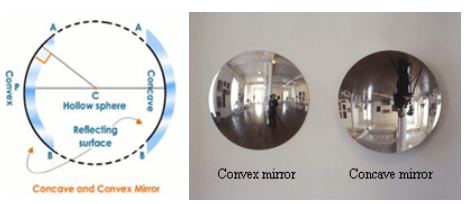Different terms associated with spherical mirrors are;

Pole (P): The centre of the spherical mirror.

Centre of curvature (C): The centre of the sphere, of which the mirror is a part.

Principal focus (F): The point on the principal axis, on which all parallel rays meet after reflection.

Radius of curvature (R): The distance between pole and centre of curvature.

Focal length (f): The distance between pole and principal focus.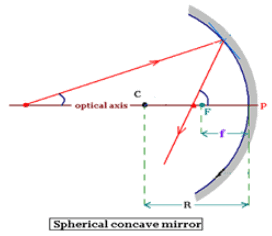Concave Mirror

Concave mirrors have the reflecting surface that bulges inward. They are also called converging mirrors because it converges all parallel beam of light incident on it. Unlike a flat mirror, concave mirrors can form real images that are projected out in front of the mirror at the place where the light focuses. Concave mirrors can be used in satellite dishes, vehicle headlights, astronomical telescopes and many more areas.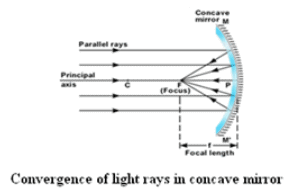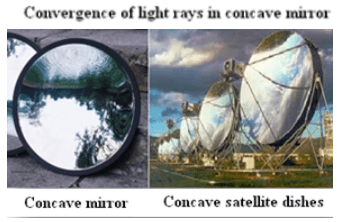Mirror Formula

The equation connecting the distance between mirror and object (u), distance between mirror and image (v), and the focal length of the mirror (f) is called mirror formula.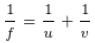Or ; The focal length of the concave mirror,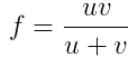Focal length by graphical method

From u-v graph :

We can measure the focal length of the given concave mirror graphically by plotting graph between u and v. For this, plot a graph with u along X axis and v along Y axis by taking same scale for drawing the X and Y axes. A curve is obtained. The point at which the bisector meets the curve gives the radius of curvature (R).

Now focal length can be calculated from the relation, R = 2f.

From 1/u – 1/v graph :

We can also measure the focal length by plotting graph between 1/-u and 1/v. Plot a graph with 1/u along X axis and 1/v along Y axis by taking same scale for drawing the X and Y axes. The graph is a straight line intercepting the axes at A and B.

The focal length can be calculated by using the relations, OA=OB= 1/f.

Learning Outcomes

• Students understand different types of mirrors and their image properties.
• Students learn and apply the mirror formula.
• Students understand the different terms associated with spherical mirrors.
• Students recall the applications of concave mirror.

Materials Required

• Concave mirror
• Stand
• Screen
• Illuminated wire gauze.
• Metre scale

Real lab Procedure

By distant object method :

• Fix the given concave mirror on the stand and place it on a table, facing towards a distant object.
• Arrange the screen on the table so that the image of the distant object is obtained on it.
• Measure the distance between mirror and screen using a metre scale. It can be taken as the focal length (f) of the mirror.

By u-v method :

• Using the focal length obtained by distant object method set the values of u (distance between mirror and object) ranging from 1.5f to 2.5f. Divide the range into a number of equal steps.
• Place the mirror in front of an illuminated wire gauze. It acts as the object.
• Now, fix the mirror at the distance u (which is obtained as 1.5f) from the wire gauze.
• Place the screen on the table facing the mirror in such a way that the reflected image lies on the screen.
• We can adjust the position of the screen to get the clear image of the wire gauze.
• Keeping the distance between object and mirror fixed, adjust the position of screen in order to get the clear image of the object.
• Measure the distance between mirror and wire gauze, as well as mirror and screen. Take these values as u and v respectively.
• Record the values of u and v in a tabular column.
• Calculate the focal length of the given concave mirror by using the relation, f = uv/(u+v).
• Repeat the experiment for different values of u (up to 2.5f) and in each time, measure v and record it in the tabular column.
• Calculate the focal length (f) of the concave mirror each time.
• Calculate the mean of all focal lengths to get the correct focal length of the given concave mirror.
• The focal length of the mirror can also be measured graphically by plotting graphs between u & v, and 1/u & 1/v.
• We can repeat the experiment with concave mirrors of different focal lengths.

Simulator Procedure (as performed through the Online Labs)

• Select the focal length using the slider.
• Click on the ‘Light On/Off’ button to turn On/Off the wire gauze.
• Select the distance between the mirror and the object (u) using the slider.
• Change the distance between the mirror and the screen (v) suing the slider to get a clear image of the wire gauze on the screen.
• You can calculate the focal length of the mirror using the equation,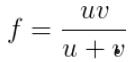• You can verify your result by clicking on the ‘Show result’ button.
• To redo the experiment, click the ‘Reset’ button.

Calculations:

Calculate the value of focal length (f) each time and find its mean.

Plot a graph with u along X axis and v along Y axis by taking same scale for drawing the X and Y axes. Draw the bisector OA and join OC and OB. Thus, OC=OB= 2f. Calculate the focal length from this.

Plot a graph with 1/u along X axis and 1/v along Y axis by taking same scale for drawing the X and Y axes. The graph is a straight line intercepting the axes at A and B. Then OA=OB= 1/f. Calculate the focal length from this.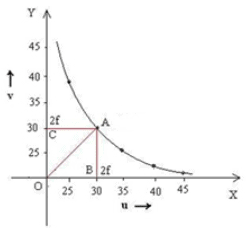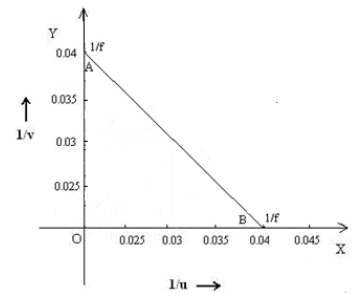u -v Graph
1/u - 1/v Graph

From u-v graph,

OB =….....….cm

OC = ...……..cm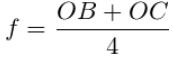=  ........... cm

From 1/u – 1/v graph,

OA =…………cm

OB =…...…... cm

Offer running on EduRev: Apply code STAYHOME200 to get INR 200 off on our premium plan EduRev Infinity!

## Physics Class 12

204 videos|288 docs|125 tests

,

,

,

,

,

,

,

,

,

,

,

,

,

,

,

,

,

,

,

,

,

,

,

,

;